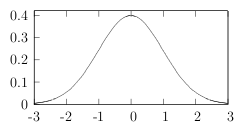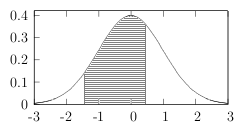gnuplot question

%% FILE: basic.tex
\documentclass[letterpaper,12pt]{article}
\begin{document}
\input{graph}
\end{document}

The file graph.tex is generated using Gnuplot like this:

#!/usr/bin/gnuplot
## FILE: graph.gnuplot
set xrange [-3:3]
set yrange [0:.42]
set ytics nomirror autofreq 0, .1
set terminal latex size 3,1.5
set output 'graph.tex'
f(x) = .39894228040143267794 * exp(-0.5 * (x ** 2))
set style line 1 linecolor rgb "black" linewidth 1
plot f(x) ls 1 notitle

Here's the execution:

$gnuplot graph.gnuplot$ pdflatex basic.tex > /dev/null
\$ evince basic.pdf &

This makes a really professional-looking graph I can put in a document.Now here's what I want to see:How do I do this with Gnuplot?